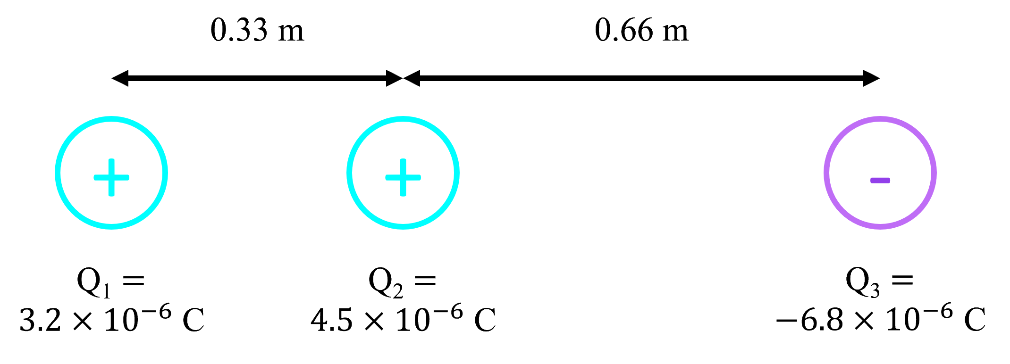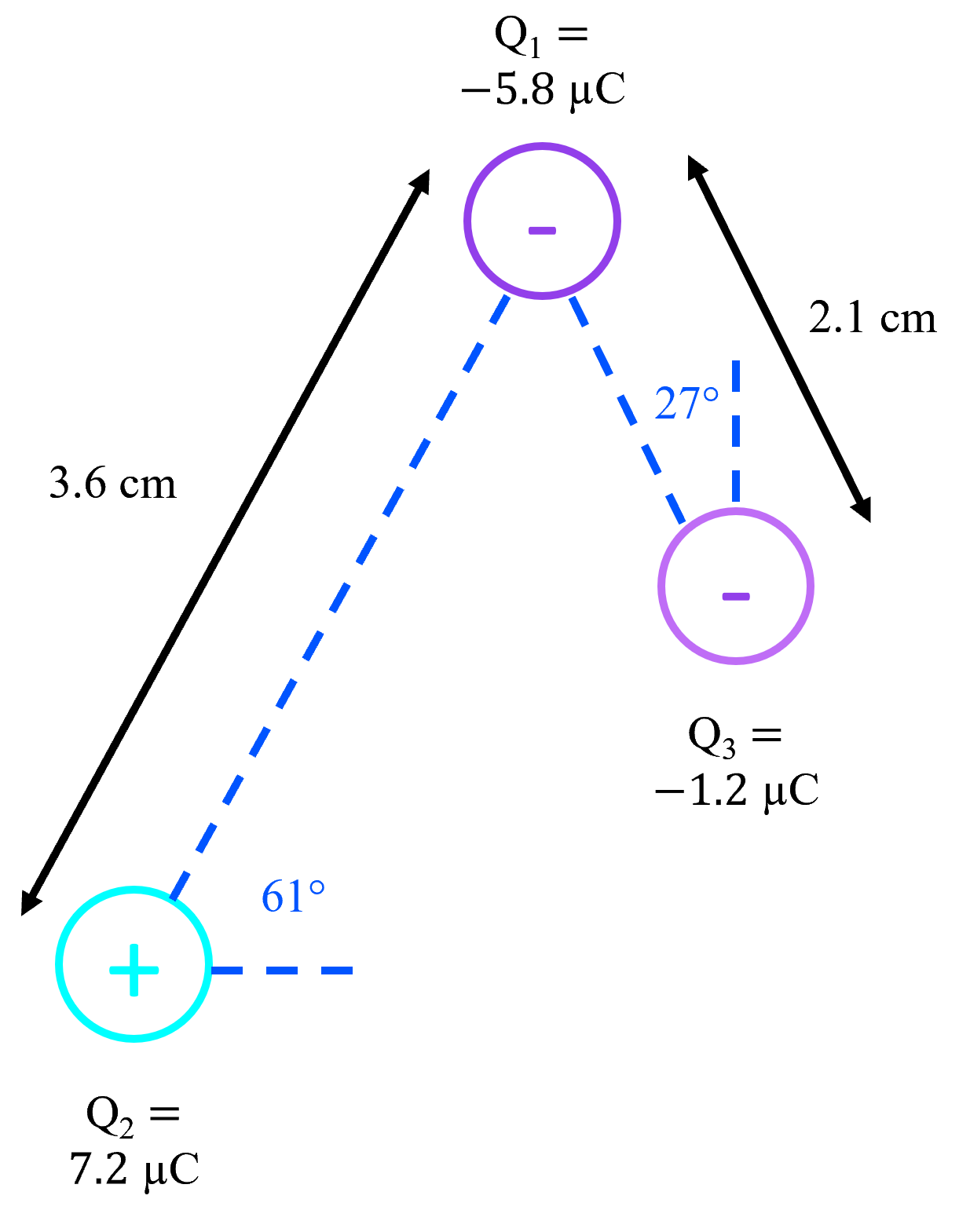# Electric force

### Electric force

#### Lessons

In this lesson, we will learn:
• Coulomb's law, which gives the electric force that one charged object exerts on another
• Calculating electric force for different arrangements of charges
Notes:

• Electrostatics deals with electric charges that are at rest ("static")
• Charge is a scalar quantity. It can be positive or negative. The positive or negative character of a charge is called polarity.
• Like gravity, electric forces act at a distance. Unlike gravity, which always pulls objects together, electric forces can either push apart or pull together charges.
• Like charges (both positive or both negative) will repel each other
• Opposite charges (one positive and one negative) will attract each other.
• Coulomb's law describes electric force (Fe).

• $|F_e| = k \frac{|Q_1 Q_2|}{r^2}$

• k (Coulomb's constant) is an experimentally determined constant that relates the size of the charges (Q1 and Q2) and radius (r¸ distance between charges) to the magnitude Fe.
• Coulomb's law only gives the magnitude of Fe and not the direction, indicated by the absolute value sign on |Fe|. Notice that k, Q, and $r^2$ are all scalars: there are no vectors on that side of the equation that could give Fe a direction. The direction of Fe must be found by considering if the charges involved would be attracted or repelled, based on their polarities.

Coulomb's Law (Electric Force)

$|F_e| = k \frac{|Q_1 Q_2|}{r^2}$
$|F_e|:$ magnitude of electric force, in newtons (N)
$k:$ Coulomb's law constant, $9.00 \times 10^9 N\centerdot m^2 / C^2$
$|Q_1|, |Q_2|:$ magnitude of each charge, in coulombs (C)
$r:$ distance between charges, in meters (m)

• 1.
Electric force in one dimension
Three point charges are fixed in positions as shown. Find the net force acting on $Q_1$.• 2.
Electric force in two dimensions
Three point charges are fixed in positions as shown. Find the net force acting on $Q_1$.